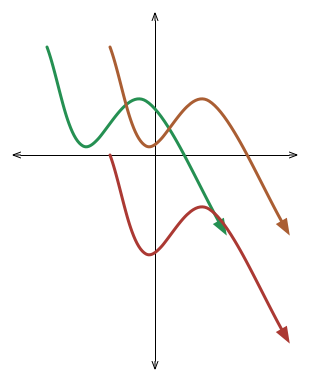# Translating across

Algebra Level 2If the $\color{#20A900} {\text{green}}$ graph is $y = f(x)$, what is the equation of the $\color{#D61F06} {\text{red}}$ graph?

The $\color{#20A900} {\text{green}}$ graph is translated 1 unit to the right, to obtain the $\color{#EC7300} {\text{orange}}$ graph.
The $\color{#EC7300} {\text{orange}}$ graph is translated 2 units down, to obtain the $\color{#D61F06} {\text{red}}$ graph.

×

Problem Loading...

Note Loading...

Set Loading...